Problem C3.4. Heaving and Pitching Airfoil in Wake

Overview

This problem is aimed at testing the accuracy and performance of high-order flow solvers for problems with deforming domains. An oscillating cylinder produces vortices that interact with an airfoil performing a typical flapping motion. The time histories of the drag coefficient on both the cylinder and on the airfoil are used as metrics, and two test cases corresponding to different stream-wise positions of the airfoil are studied.

Governing Equations

The governing equations for this problem are the 2D compressible Navier-Stokes equations with a constant ratio of specific heats equal to 1.4 and a Prandtl number of 0.72.

Flow Conditions

The free-stream has a mach number () of 0.2 and an angle of attack (a) of. The Reynolds number based on the chord of the airfoil is 1000 (or, equivalently, 500 based on the diameter of the cylinder).

Geometry

The geometry consists of a cylinder of diameter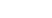centered at the origin, and a NACA0012 airfoil with chord length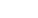positioned downstream from the cylinder. The geometry is given by the modification of thecoefficient to give zero trailing edge thickness: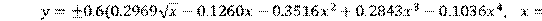The center of the cylinder and the 1/3 chord of the airfoil are separated by a distance. Both bodies are heaving in an oscillating motion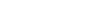, where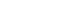and. In addition, the airfoil it pitching about its 1/3 chord by an angle, where the amplitudeand the phase shift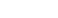.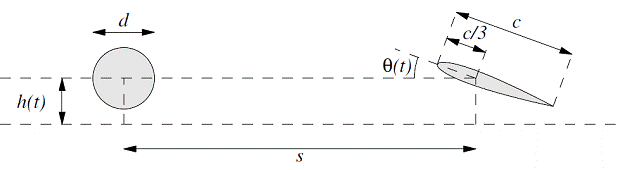Requirements

1.     Perform the indicated simulation to an elapsed time of 100 time units (or 20 periods), for the two test cases (a)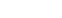and (b)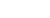. Maintain a time history of the drag coefficients on the cylinder and on the airfoil, and calculate the time-averaged drag coefficients for the last (10th) period only. Perform a grid/timestep convergence study to get the time-averaged values accurate to within 1 drag count. Record the work units.

2.     Provide the work units, the converged time history of lift and drag on the wing, nDOFs in the solution, and the distance to the far field boundary for each case. Submit this data to the workshop contact.